## Gaussian Integral

The Gaussian integral, also called the Probability Integral, is the integral of the 1-D Gaussian over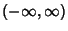. It can be computed using the trick of combining two 1-D Gaussians(1)

and switching to Polar Coordinates,(2)

However, a simple proof can also be given which does not require transformation to Polar Coordinates (Nicholas and Yates 1950).

The integral from 0 to a finite upper limitcan be given by the Continued Fraction(3)

The general class of integrals of the form(4)

can be solved analytically by setting(5)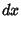(6)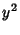(7)

Then(8)

For, this is just the usual Gaussian integral, so(9)

For, the integrand is integrable by quadrature,(10)

To compute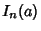for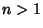, use the identity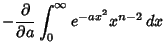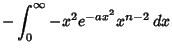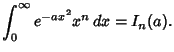(11)

ForEven,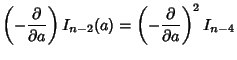(12)

so(13)

If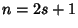is Odd, then(14)

so(15)

The solution is therefore(16)

The first few values are therefore(17)(18)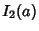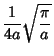(19)(20)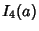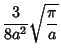(21)(22)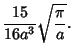(23)

A related, often useful integral is(24)

which is simply given by(25)

References

Nicholas, C. B. and Yates, R. C. The Probability Integral.'' Amer. Math. Monthly 57, 412-413, 1950.